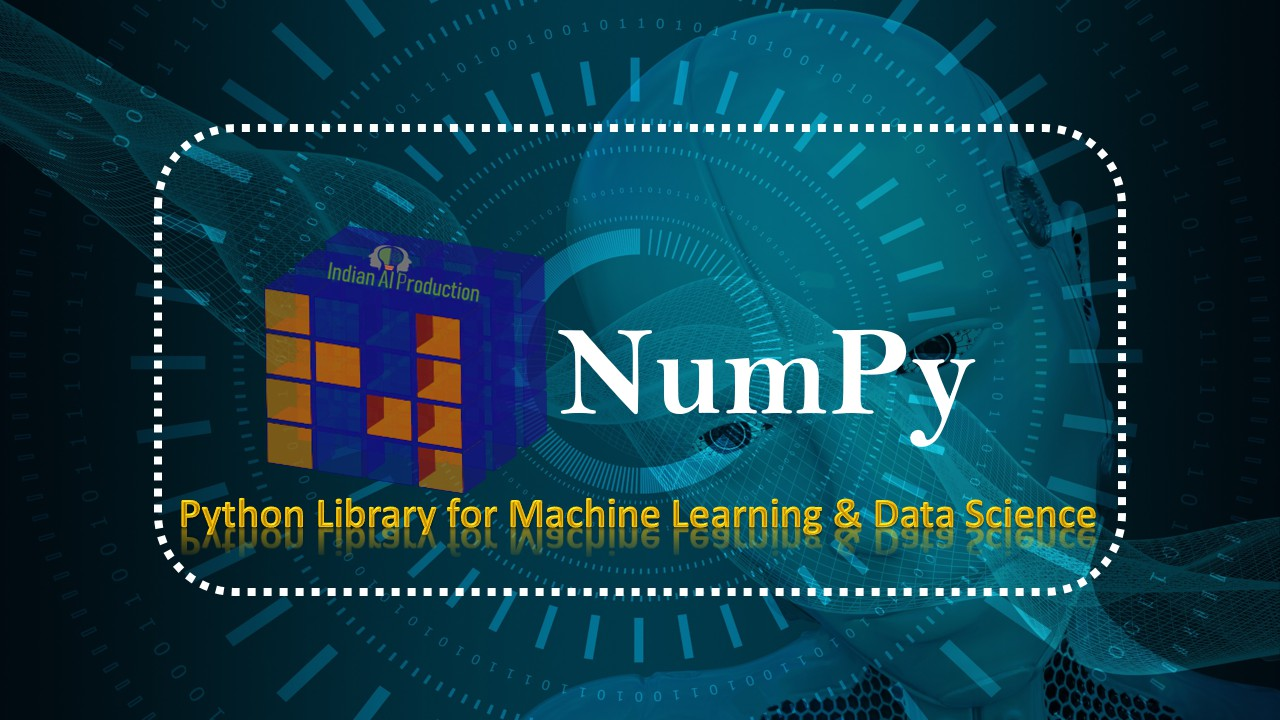## NumPy String Operations | Python NumPy Tutorial

If you want to work on string data then NumPy string operations methods help to do work easy. The python NumPy support a bunch of string operations, string comparison, and string information methods. So lets start with Python NumPy String Operations Methods To start the use of string methods need to import NumPy package and some raw string data. import numpy as np # import numpy package ch_name...
Top